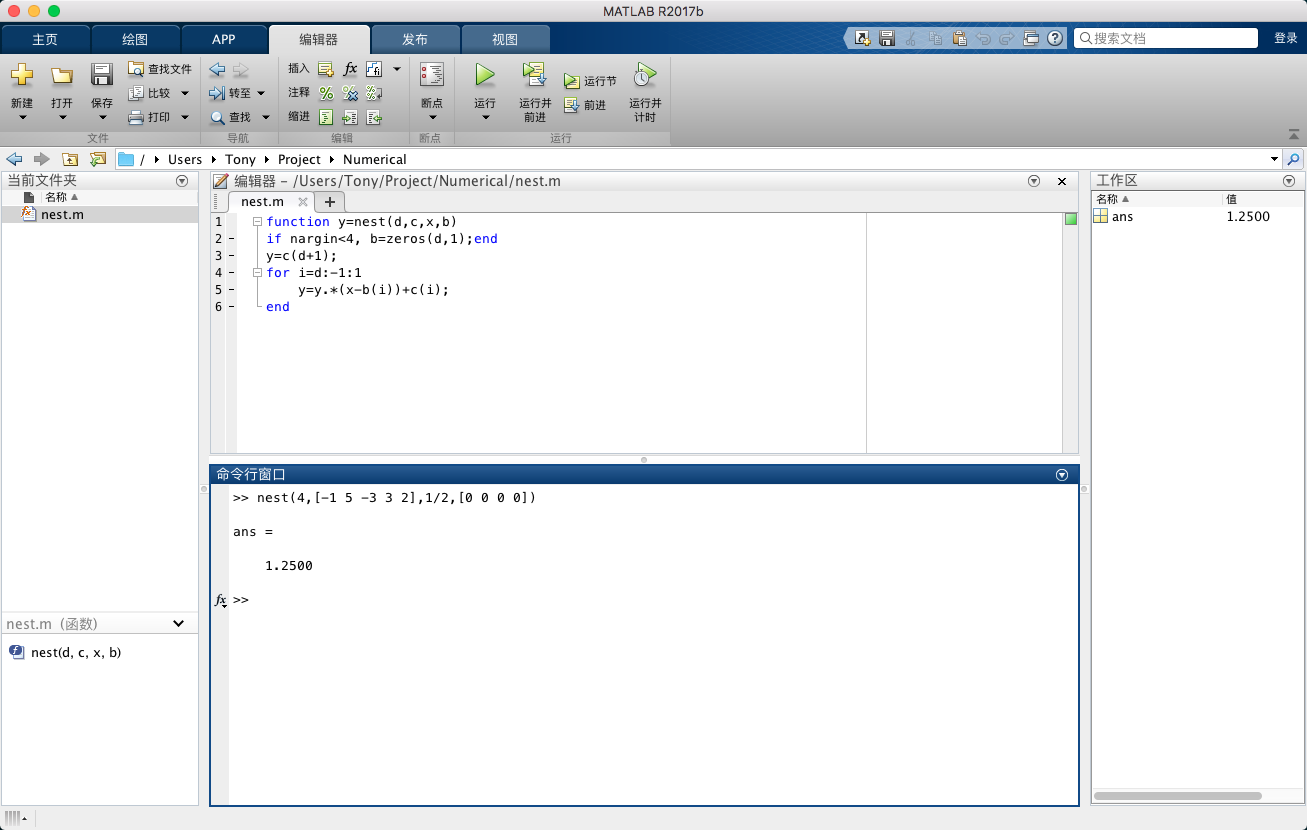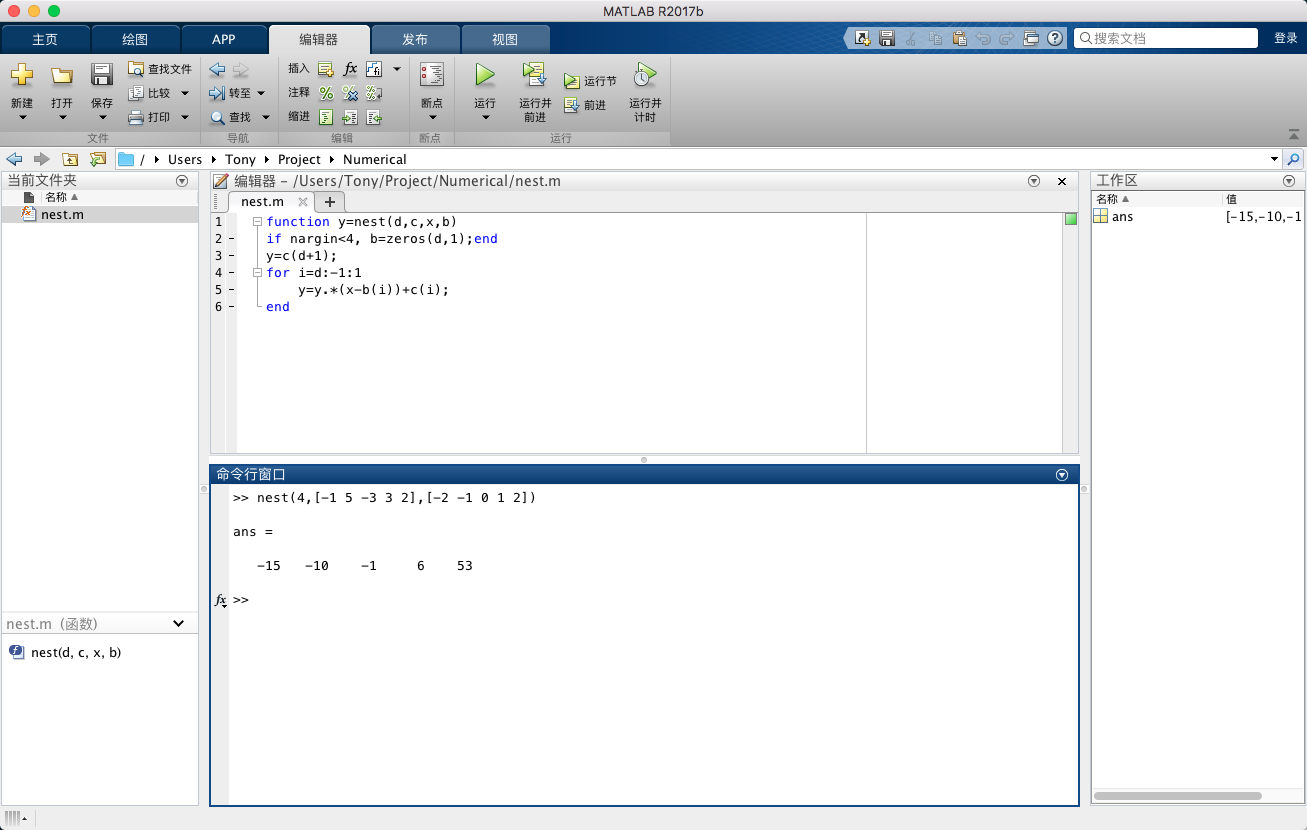Abstract: 本文介绍多项式求值的相关内容。
Keywords: 多项式求值，霍纳方法

# 多项式求值

## 三种方法

$$P(x)=2x^4+3x^3-3x^2+5x-1$$

$$P(\frac{1}{2})=2\times \frac{1}{2}\times \frac{1}{2}\times \frac{1}{2}\times \frac{1}{2} +3\times \frac{1}{2}\times \frac{1}{2}\times \frac{1}{2} -3\times \frac{1}{2}\times \frac{1}{2} +5\times \frac{1}{2}-1$$

$$x^4=x\times x^3\\ x^3=x\times x^2\\ \vdots$$

$$\frac{1}{2}\times \frac{1}{2}$$

$$\frac{1}{2}\times \frac{1}{2}\times \frac{1}{2}=\frac{1}{2}\times a$$

\begin{aligned} P(x) &=2x^4+3x^3-3x^2+5x-1\\ &=-1+x(5-3x+3x^2+2x^3)\\ &=-1+x(5-x(3+3x+2x^2))\\ &=-1+x(5-x(3+x(3+2x)))\\ \end{aligned}

## 深入理解这个例子

• 计算机在简单计算的时候速度极快
• 简单计算会被多次重复执行，所以高效的简单计算非常重要
• 最好的计算方法并不是最显而易见的那种。

20世纪后五十年，结合计算机硬件，常见问题已经开发出了许多有效的求解方法（也就是利用计算机帮我们高效的完成数学计算）。

$$c_1+x(c_2+x(c_3+x(\dots)))$$

$$c_1+(x-r_1)(c_2+(x-r_2)(c_3+(x-r_3)(\dots)))$$

## 编程实现Matlab的代码比较简单，虽然我们写过matlab的代码，但是这段似乎挺简单，但是这语法，写C太久，看别的语言总感觉语法混乱/(ㄒoㄒ)/~~

Matlab还可以同时输入多个 $x$ 真的很神奇 😓$$P(x)=1+x(\frac{1}{2}+(x-2)(\frac{1}{2})+(x-3)(-\frac{1}{2}))$$

0%